双样本 Poisson 率 的方法和公式

统计量

Minitab 根据样本生成下面的描述性统计量。只有在将“长度”改为默认值 1 以外的值时，Minitab 才显示均值。

针对正态近似的率差值的假设检验

公式

Minitab 将下面的 p 值等式用于各自的备择假设：

表示法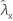样本 X 的率的观测值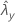样本 Y 的率的观测值
ζ 两个样本的总体率之间差值的实际值
ζ0 两个样本的总体率之间差值的假设值
m 样本 X 的样本数量
n 样本 Y 的样本数量
tx 样本 X 的长度
ty 样本 Y 的长度

针对精确方法的率差值的假设检验

公式

H0ζ = λxλy = 0，或者 H0λx = λy

S | W ~ Binomial(w, p)

W = S + U

Minitab 将下面的 p 值等式用于各自的备择假设：
• H1ζ > 0：p 值 = P(S s | w = s + u, p = p0)

• H1ζ < 0：p 值 = P(S s | w = s + u, p = p0)

• H1ζ ≠ 0：
• 如果 P(S s | w = s + u, p = p0) ≤ 0.5，或者 P(S s | w = s + u, p = p0) ≤ 0.5

则 p 值 = 2 × min {P(S s | w = s + u, p = p0)，P(S s | w = s + u, p = p0)}

• 否则，p 值 = 1.0

表示法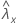样本 X 的率的观测值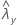样本 Y 的率的观测值
λx总体 X 的率的实际值
λy总体 Y 的率的实际值
ζ两个样本的总体率之间差值的实际值
tx样本 X 的长度
ty样本 Y 的长度
m样本 X 的样本数量
n样本 Y 的样本数量

针对合并率方法的率差值的假设检验

公式

Minitab 将下面的 p 值等式用于各自的备择假设：

表示法样本 X 的率的观测值样本 Y 的率的观测值
λx总体 X 的率的实际值
λy总体 Y 的率的实际值
ζ两个样本的总体率之间差值的实际值
m样本 X 的样本数量
n样本 Y 的样本数量
tx样本 X 的长度
ty样本 Y 的长度

针对正态近似方法的均值差值的假设检验

公式

Minitab 将下面的 p 值等式用于各自的备择假设：

表示法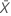样本 X 中平均发生次数的观测值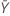样本 Y 中平均发生次数的观测值
δ 两个样本的总体均值之间差值的实际值
δ 0 两个样本的总体均值之间差值的假设值
m 样本 X 的样本数量
n 样本 Y 的样本数量

针对精确方法的均值差值的假设检验

公式

S | W ~ Binomial(w, p)

W = S + U

Minitab 将下面的 p 值等式用于各自的备择假设：

H1δ > 0：p 值 = P(S s | w = s + u, δ = 0)

H1δ < 0：p 值 = P(S s | w = s + u, δ = 0)

H1δ ≠ 0：
• 如果 P(Ss|w = s + u, δ = 0) ≤ 0.5

或者 P(Ss|w = s + u, δ = 0) ≤ 0.5

则：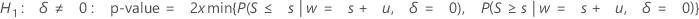• 否则，p 值 = 1.0

表示法

μx总体 X 中平均发生次数的实际值
μy总体 Y 中平均发生次数的实际值
δ两个样本的总体均值之间差值的实际值
m样本 X 的样本数量
n样本 Y 的样本数量

针对合并均值方法的均值差值的假设检验

公式

Minitab 将下面的 p 值等式用于各自的备择假设：

表示法样本 X 中平均发生次数的观测值样本 Y 中平均发生次数的观测值
µx总体 X 中平均发生次数的实际值
µy总体 Y 中平均发生次数的实际值
δ两个样本的总体均值之间差值的实际值
m样本 X 的样本数量
n样本 Y 的样本数量

率差值的置信区间

表示法样本 X 的率的观测值样本 Y 的率的观测值
ζ两个样本的总体率之间差值的实际值
zx标准正态分布的 x 百分位点上限，其中 0 < x < 1
m样本 X 的样本数量
n样本 Y 的样本数量
tx样本 X 的长度
ty样本 Y 的长度

率差值的置信界限

表示法样本 X 的率的观测值样本 Y 的率的观测值
ζ两个样本的总体率之间差值的实际值
zx标准正态分布的 x 百分位点上限，其中 0 < x < 1
m样本 X 的样本数量
n样本 Y 的样本数量
tx样本 X 的长度
ty样本 Y 的长度

均值差值的置信区间

表示法样本 X 中平均发生次数的观测值样本 Y 中平均发生次数的观测值
δ两个样本的总体均值之间差值的实际值
zx标准正态分布的 x 百分位点上限，其中 0 < x < 1
m样本 X 的样本数量
n样本 Y 的样本数量

均值差值的置信界限

表示法样本 X 中平均发生次数的观测值样本 Y 中平均发生次数的观测值
δ两个样本的总体均值之间差值的实际值
zx标准正态分布的 x 百分位点上限，其中 0 < x < 1
m样本 X 的样本数量
n样本 Y 的样本数量